1592510520

# Exchange Rate Prediction: Time Series Forecasting with ARIMA

Here comes the final part of my end-to-end project in Data Science. If you have followed or read the previous two parts, you might have known what to expect in this article. But if you haven’t, do not hesitate to check them out: 1st part and 2nd part.
Here’s a summary of the entire structure of this project:
Part 1: Explanatory Data Analysis (EDA) & Data Visualisation (Bonus: Hypothesis Testing)
Part 2: Machine Learning with 4 Regression Models
Part 3: Machine Learning (cont.) with ARIMA
With the first part paving the foundation for the analysis with data cleaning and visualization and the second employing Regression models to fit all data points, this final part will utilize them all to predict the future (in this case, AUD/USD exchange rates in 2020). In order to achieve so, prerequisites and process should be taken into account:

#time-series-analysis #stationary #data-science #python #machine-learning

## Buddha Community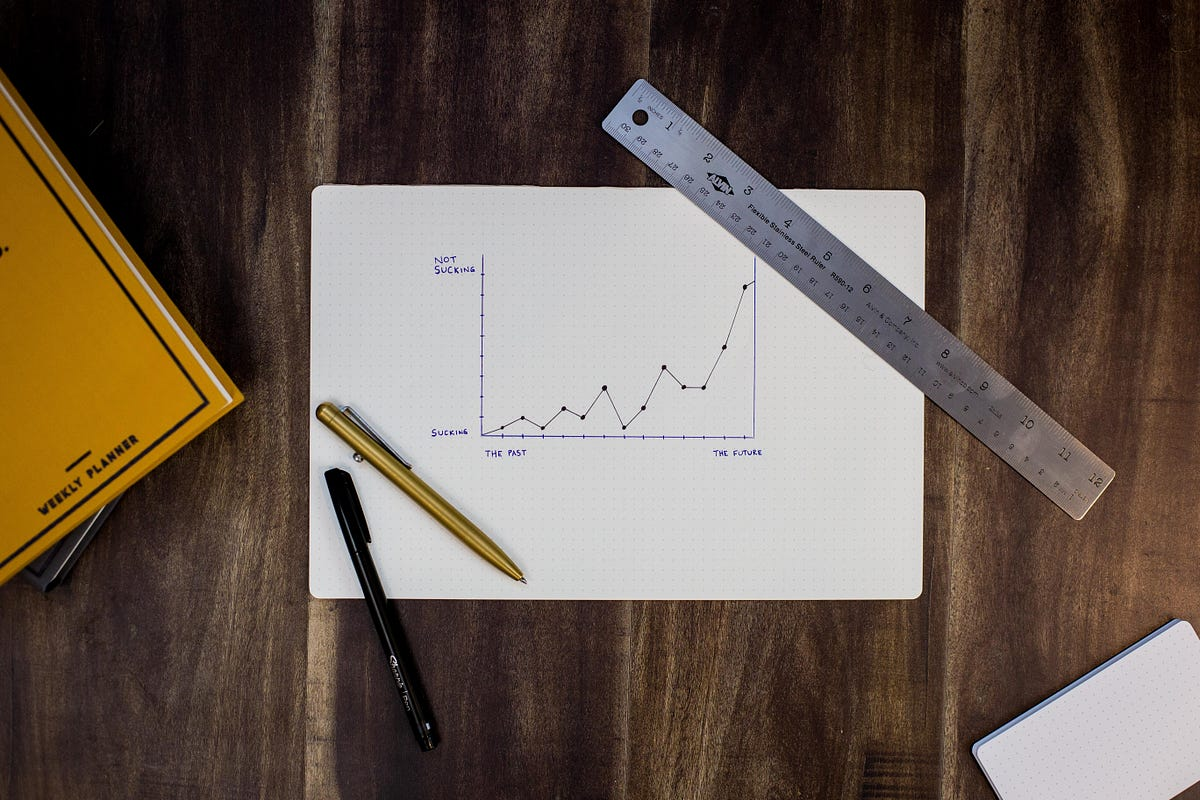1623226129

## Time Series Analysis Using ARIMA Model With Python

Time series_ is a sequence of time-based data points collected at specific intervals of a given phenomenon that undergoes changes over time. In other words, time series is a sequence taken at consecutive equally spaced points in the time period._

As a example, we can present few time series data sets in different domains such as pollution levels, Birth rates, heart rate monitoring, global temperatures and Consumer Price Index etc. At the processing level, above datasets are tracked, monitored, down sampled, and aggregated over time.

There are different kind of time series analysis techniques in the big data analytical field. Among them few are,

• Autoregression (AR)
• Moving Average (MA)
• Autoregressive Moving Average (ARMA)
• Autoregressive Integrated Moving Average (ARIMA)
• Seasonal Autoregressive Integrated Moving-Average (SARIMA)

ARIMA Model

ARIMA Model is simple and flexible enough to capture relationship we would see in the data and It aims to explain the autocorrelation between the data points using past data. We can decompose the ARIMA model as follow to grab the key elements of it.

• **AR: _Auto regression. _**This is a model that uses the dependent relationship between the data and the lagged data.
• **I:_ Integrated. _**The use of differencing of raw observations (e.g. subtracting an observation from an observation at the previous time step) in order to make the time series stationary.
• **MA: _Moving average. _**A model that uses the relationship between the observations and the residual error from the moving average model applied to lagged observations.

Dataset Explanation

Exploratory Analysis

#python #time-series-analysis #pandas #forecasting #arima #time series analysis using arima model with python1595685600

## What is Time Series Forecasting?

In this article, we will be discussing an algorithm that helps us analyze past trends and lets us focus on what is to unfold next so this algorithm is time series forecasting.

What is Time Series Analysis?

In this analysis, you have one variable -TIME. A time series is a set of observations taken at a specified time usually equal in intervals. It is used to predict future value based on previously observed data points.

Here some examples where time series is used.

2. Understand the past behavior
3. Plan future
4. Evaluate current accomplishments.

Components of time series :

1. Trend: Let’s understand by example, let’s say in a new construction area someone open hardware store now while construction is going on people will buy hardware. but after completing construction buyers of hardware will be reduced. So for some times selling goes high and then low its called uptrend and downtrend.
2. **Seasonality: **Every year chocolate sell goes high during the end of the year due to Christmas. This same pattern happens every year while in the trend that is not the case. Seasonality is repeating same pattern at same intervals.
3. Irregularity: It is also called noise. When something unusual happens that affects the regularity, for example, there is a natural disaster once in many years lets say it is flooded so people buying medicine more in that period. This what no one predicted and you don’t know how many numbers of sales going to happen.
4. Cyclic: It is basically repeating up and down movements so this means it can go more than one year so it doesn’t have fix pattern and it can happen any time and it is much harder to predict.

Stationarity of a time series:

A series is said to be “strictly stationary” if the marginal distribution of Y at time t[p(Yt)] is the same as at any other point in time. This implies that the mean, variance, and covariance of the series Yt are time-invariant.

However, a series said to be “weakly stationary” or “covariance stationary” if mean and variance are constant and covariance of two-point Cov(Y1, Y1+k)=Cov(Y2, Y2+k)=const, which depends only on lag k but do not depend on time explicitly.

#machine-learning #time-series-model #machine-learning-ai #time-series-forecasting #time-series-analysis1596999420

## A Real-World Time Series Data Analysis and Forecasting

The stationarity of a time series data means that the statistical properties like mean, variance, and autocorrelation of the series do not change over time. The notion of stationarity of a series is important for applying statistical forecasting models since:

1. most of the statistical methods like ARIMA are based on the assumption that the process is stationary or approximately stationary .
2. a stationary time series can provide meaningful sample statistics like mean, variance, correlation with other variables .

The stationarity of the process can be verified by visually check the time series plot or variogram of the series. Statistical tests like the** Augmented Dickey-Fuller** test can be performed to check the stationarity of a process. In this article verify the stationarity by visually check the time series plot and variogram.

**Time series plot — **A given time series plot can be considered as a stationary process if it shows constant mean and variance over the period of time.

**Variogram — **is a graphical tool to check the stationarity of a time series data. If the variogram of a given process (time series) shows stability after a certain number of lags, then the process is defined to be a stationary process.

If the original time series does not show stationarity then it can be stabilized by implementing transformation (e.g. log transformation) and differencing the series.

We will apply the ARIMA model to a real-world dataset “Daily Average Exchange Rates Between US Dollars and Euro”. The dataset is given in the book “Time Series Analysis and Forecasting by Example” by Sorren Bissgard and Murat Kulachi. A snippet of the dataset is given below: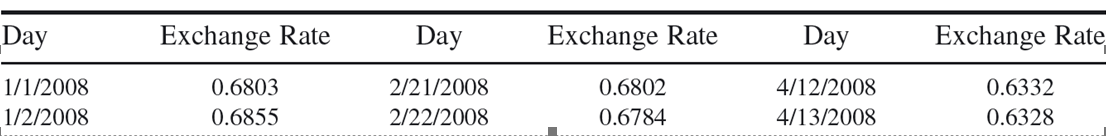Daily Average Exchange Rates Between US Dollars and Euro

Stationarity: Original time series and its’ Variogram

Figure 1 and 2 illustrates the original time series and its variogram, respectively. Fig. 1 shows that the series is not stationary as it does not follow constant mean and variance. The variogram in Fig. 2 does not show stability as after around 80 lags it shows a decreasing trend and in the long run, it may not able to maintain a stable pattern which indicates the process is not stationary.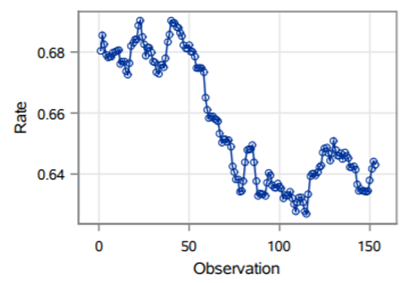Fig. 1: Original time series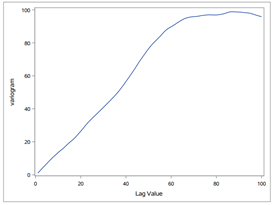Fig. 2: Variogram of the original series

Stationarity: Differencing the original series

Figure 3 and 4 illustrates the time series of the one differenced process of the original series and its variogram, respectively. Fig. 3 shows that the one differenced series follow constant mean and variance indicating a stationary series. Additionally, the first differenced variogram in Fig. 4 shows the characteristics of stationary series as it demonstrates settling down in the long run. Hence, the one differenced series would be appropriate to be used for further analysis.

#arima #time-series-forecasting #real-world-data #time-series-analysis #stationarity #data analysis1598640600

## Naive Forecast, Moving Average

Let’s employ some basic statistical methods to predict stick prices. We will first learn what these methods mean followed by quick code implementations. You’ll be surprised to see that such simple approaches have great accuracies!

This is our second blog under Stock Price Prediction. Our first blog in this series provides an easy-to-understand guide to Facebook Prophet, a Pretrained Model to Forecast Time Series.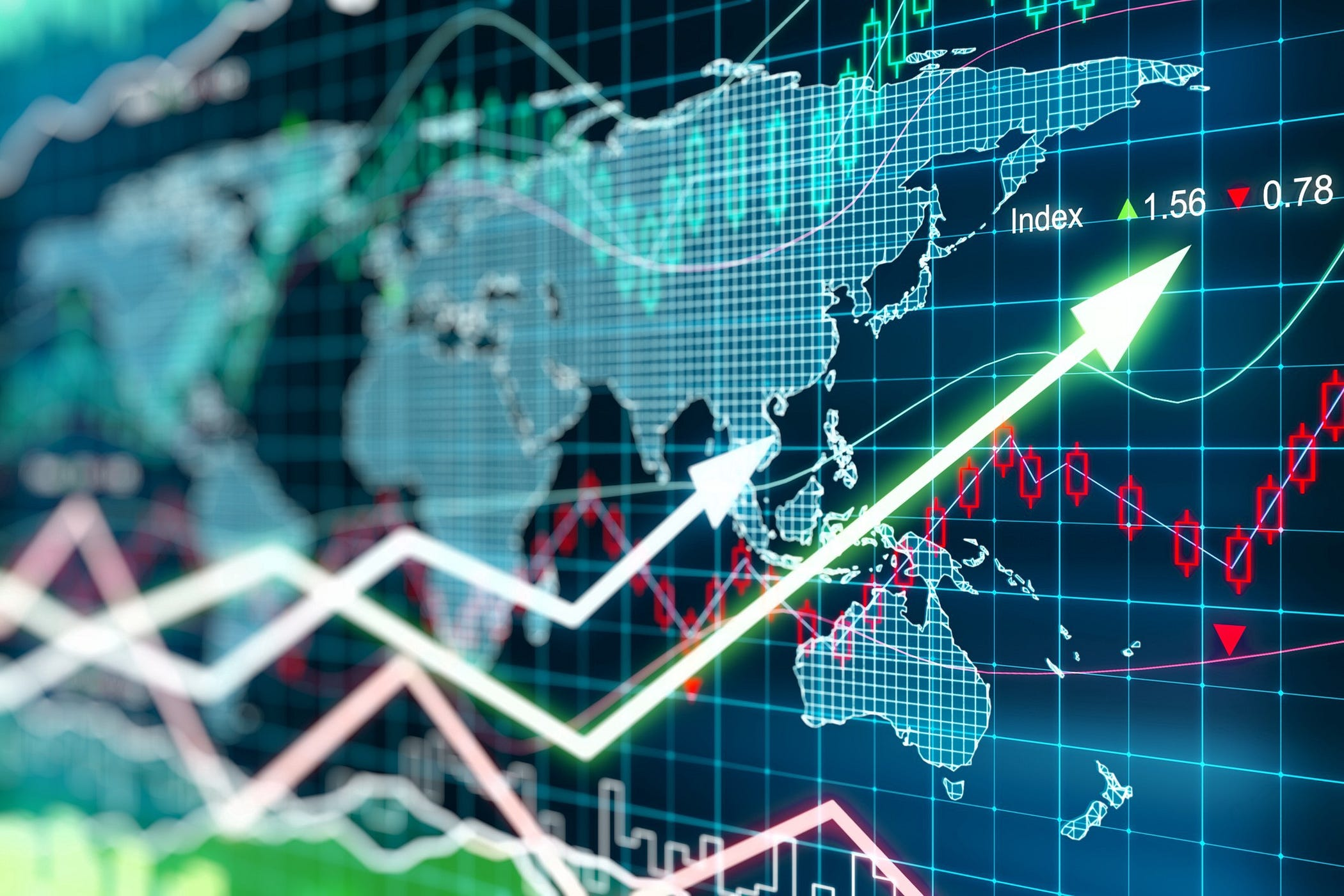## Naive Forecast

Naive Forecast is the most basic method of forecasting stock prices. This approach preaches that the forecast is nothing but the value of the variable at a previous timestamp.

For instance, in a dataset where the timestamp is a day, the predicted opening stock price for tomorrow is simply today’s opening value. Though simple, it yields awesome results! Try for yourself!

The reason Naive Forecast works so well is because variables like stock price highly depend on their values in the past. Since sudden changes in the prices of the stock is unlikely. The previous day’s value is usually very close to the following day’s value.

However, the reason this method isn’t widely used is that most of the time, we’d like to predict stock price values for a number of days in the future rather than a single day. This method cannot be used in such cases.

#time-series-forecasting #forecast #time-series-analysis #statistics #stock-prediction #data analysis1619629200

## ARIMA Model in Python – Predictive Hacks

ARIMA is one of the most popular satistical models. It stands for AutoRegressive Integrated Moving Average and it’s fitted to time series data either for forecasting or to better understand the data. We will not cover the whole theory behind the ARIMA model but we will show you what’s the steps you need to follow to apply it correctly.

They key aspects of ARIMA model are the following:

• AR: Autoregression.  This indicates that the time series is regressed on its own lagged values.
• I: Integrated.   This indicates that the data values have been replaced with the difference between their values and the previous values in order to convert the series into stationary.
• **MA: Moving Average. **This indicates that the regression error is actually a linear combination of error terms whose values occurred contemporaneously and at various times in the past.

The ARIMA model can be applied when we have seasonal or non-seasonal data. The difference is that when we have seasonal data we need to add some more parameters to the model.

For non-seasonal data the parameters are:

• p: The number of lag observations the model will use
• d: The number of times that the raw observations are differenced till stationarity.
• q: The size of the moving average window.

For seasonal data we need to add also the following:

• P: The number of seasonal lag observations the model will use
• D: The number of times that the seasonal observations are differenced till stationarity.
• Q: The size of the seasonal moving average window.
• m: The number of observations of 1 season

#python #arima #data science #forecasting #predictions #python #time series